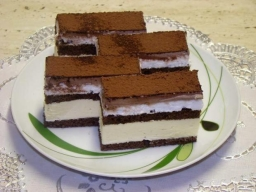# Pinwheels 13751

Mom bought desserts for her birthday party. The pinwheel cost CZK 14, and the Indian women CZK 11. She paid 201 crowns for 15 desserts. How many pinwheels did she buy, and how many Indians?

i =  3
v =  12

### Step-by-step explanation:

14v+11i = 201
v+i=15

14·v+11·i = 201
v+i=15

11i+14v = 201
i+v = 15

Row 2 - 1/11 · Row 1 → Row 2
11i+14v = 201
-0.27v = -3.27

v = -3.27272727/-0.27272727 = 12
i = 201-14v/11 = 201-14 · 12/11 = 3

i = 3
v = 12

Our linear equations calculator calculates it.Did you find an error or inaccuracy? Feel free to write us. Thank you!

Tips for related online calculators
Do you have a linear equation or system of equations and looking for its solution? Or do you have a quadratic equation?